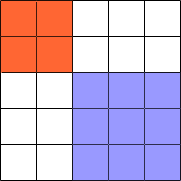Quandaries and Queries My name is Cynthia and I am a Student @University of Phoenix online taking Math208-College Math I. I am pretty bad in algebra and need to figure out the following problem:   An algebra student incorrectly used the distributive property and wrote 3(x+2) = 3x+2. How would you explain to him the correct result, without the use of the distributive law?   Explain why the square of the sum of two numbers is different from the sum of the squares of two numbers. Hi Cynthya, In both your questions I would use an example. For the first question, suppose that you went shopping and found some T-shirts on sale for \$x each. There is also a sales tax that adds \$2 to each T-shirt. You really like the shirts so you decide to buy 3 if you can afford them. So, what is it going to cost you to buy 3 shirts? It costs \$x for each shirt so 3 will cost 3\$x There is a \$2 tax on each shirt so the tax will be 3\$2 = \$6 Thus the three shirts will cost 3\$x + \$6 That is 3(x + 2) = 3x + 6. For the second problem try some numbers. (2 + 3)2 = 52 = 25 and yet 22 + 32 = 4 + 9 = 13. Another way to approach this problem is to see "square" as a geometric concept. Compare a square with sides of length 2 + 3 = 5, to squares with sides of length 2 and 3.Penny Go to Math Central